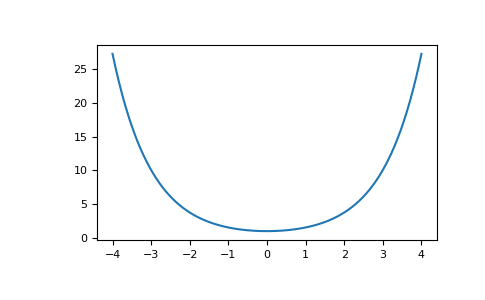numpy.sinh

numpy.tanh

# numpy.cosh¶

`numpy.``cosh`(x, /, out=None, *, where=True, casting='same_kind', order='K', dtype=None, subok=True[, signature, extobj]) = <ufunc 'cosh'>

Hyperbolic cosine, element-wise.

Equivalent to `1/2 * (np.exp(x) + np.exp(-x))` and `np.cos(1j*x)`.

Parameters: x : array_like Input array. out : ndarray, None, or tuple of ndarray and None, optional A location into which the result is stored. If provided, it must have a shape that the inputs broadcast to. If not provided or None, a freshly-allocated array is returned. A tuple (possible only as a keyword argument) must have length equal to the number of outputs. where : array_like, optional Values of True indicate to calculate the ufunc at that position, values of False indicate to leave the value in the output alone. **kwargs For other keyword-only arguments, see the ufunc docs. out : ndarray or scalar Output array of same shape as x. This is a scalar if x is a scalar.

Examples

```>>> np.cosh(0)
1.0
```

The hyperbolic cosine describes the shape of a hanging cable:

```>>> import matplotlib.pyplot as plt
>>> x = np.linspace(-4, 4, 1000)
>>> plt.plot(x, np.cosh(x))
>>> plt.show()
```